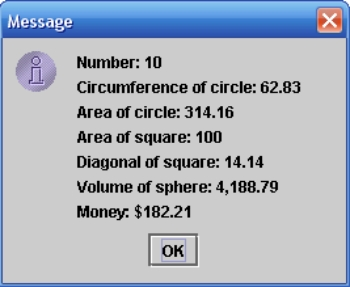### Doing Basic Math

7 points

Introduction
In this program you use a constant and some methods from the Math class to calculate some math formulas. You ask the user for a number, and then you calculate 6 quantities that depend on that number. Finally, you print out the results of these calculations. You do all of this in the method 'calculateValues()'. You also make one small change to 'main(String[] args)' -- adding a question inside the double-quotes in this line:
input = JOptionPane.showInputDialog("");

calculateValues()
In 'main()' you ask the user for a number, and you then calculate 6 quantities that are based on that number. You will need 6 local variables in 'calculateValues()' to store:

• the circumference of a circle : 2π * number
• the area of a circle : π * number2
• the area of a square : number2
• the diagonal of a square : √2  * number
• the volume of a sphere : 4./3. * π * number3
• the amount of money you'd have if you invested \$100 at a 6% interest rate for 'number' years : 100e0.06 * number

Use descriptive names like 'circleArea' for these 6 variables. This helps you and anyone else who looks at your program to understand what is going on. Here is how you would create the variable 'circleArea' inside 'calculateValues()':
double circleArea;

To use the math constant π, use 'Math.PI'. Other math constants are also available.

To take the square root of 'number,' use 'Math.sqrt(number)'. To find a number like e3, use 'Math.exp(3)'. To calculate 34, use 'Math.pow(3, 4)'.

After calculating each of the 6 values listed above, add a line of descriptive output to a String. For example, in my program I first declared a String:
String msg;
The first line of descriptive output looks like this:
msg = msg + "Number: " + f.format(number) + "\n";
or like this:
msg += "Number: " + f.format(number) + "\n";
Finally, after adding output for all 6 numbers, print out 'msg' in this way:
JOptionPane.showMessageDialog(null, msg);

Here is the box that pops up at the end of my program if I enter '10' as the number:I left this line in 'calculateValues()':
number = Math.abs(number);
This line uses the absolute value function to make sure that if the user enters a negative number, it is converted to positive before it is used.

To use Math methods like 'Math.pow()' and 'Math.sqrt(),' and Math constants like 'Math.PI,' you will need to add this line to the top of your program:
import java.lang.Math.*;

### Extra Credit

1 point

If you want to get a little fancy and set the number of decimal digits that print to 2, add this code to 'calculateValues()':
NumberFormat f = NumberFormat.getNumberInstance();
f.setMaximumFractionDigits(2);
Then whenever you print out a 'double,' put that variable inside 'f.format()'. For example, if you have a 'double' called 'mpg,' this line would cause 'mpg' to print with 2 decimal digits:
System.out.println("Miles per gallon: " + f.format(mpg) );
You also have to add the line 'import java.text.NumberFormat;' at the top of the program.